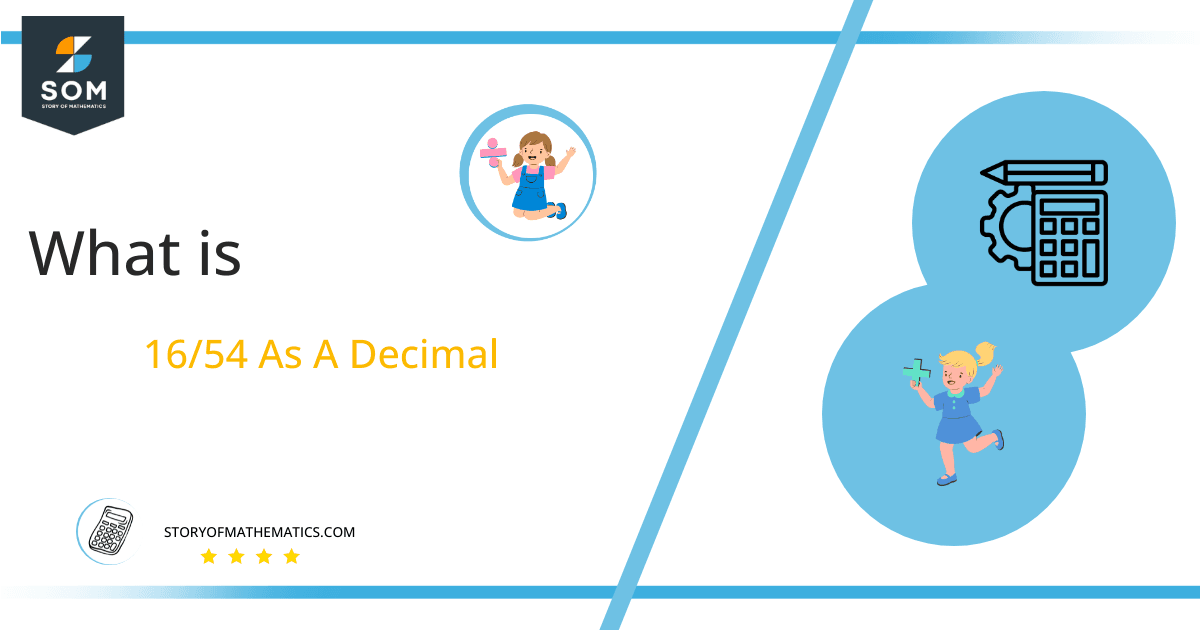# What Is 16/54 as a Decimal + Solution With Free Steps

The fraction 16/54 as a decimal is equal to 0.2962963.

Recurring decimals and  Non- recurring decimals are the types of decimal numbers. Alternative names for Non-recurring decimals are terminating decimals and Non-repeating decimals. e.g. 0.296 is a non-recurring decimal numberHere, we are more interested in the division types that result in a Decimal value, as this can be expressed as a Fraction. We see fractions as a way of showing two numbers having the operation of Division between them that result in a value that lies between two Integers.

Now, we introduce the method used to solve said fraction-to-decimal conversion, called Long Division, which we will discuss in detail moving forward. So, let’s go through the Solution of fraction 16/54.

## Solution

First, we convert the fraction components, i.e., the numerator and the denominator, and transform them into the division constituents, i.e., the Dividend and the Divisor, respectively.

This can be done as follows:

Dividend = 16

Divisor = 54

We introduce the most important quantity in our division process: the Quotient. The value represents the Solution to our division and can be expressed as having the following relationship with the Division constituents:

Quotient = Dividend $\div$ Divisor = 16 $\div$ 54

This is when we go through the Long Division solution to our problem. The following figure shows the long division:Figure 1

## 16/54 Long Division Method

We start solving a problem using the Long Division Method by first taking apart the division’s components and comparing them. As we have 16 and 54, we can see how 16 is Smaller than 54, and to solve this division, we require that 16 be Bigger than 54.

This is done by multiplying the dividend by 10 and checking whether it is bigger than the divisor or not. If so, we calculate the Multiple of the divisor closest to the dividend and subtract it from the Dividend. This produces the Remainder, which we then use as the dividend later.

Now, we begin solving for our dividend 16, which after getting multiplied by 10 becomes 160.

We take this 160 and divide it by 54; this can be done as follows:

160 $\div$ 54 $\approx$ 2

Where:

54 x 2 = 108

This will lead to the generation of a Remainder equal to 160 – 108 = 52. Now this means we have to repeat the process by Converting the 52 into 520 and solving for that:

520 $\div$ 54 $\approx$ 9

Where:

54 x 9 = 486

This, therefore, produces another Remainder equal to 520 – 486 = 34. Now we must solve this problem to the Third Decimal Place for accuracy, so we repeat the process with dividend 340.

340 $\div$ 54 $\approx$ 6

Where:

54 x 6 = 324

Finally, we have a Quotient generated after combining the three pieces of it as 0.296=z, with a Remainder equal to 16.Images/mathematical drawings are created with GeoGebra.